Next: Learning Module Exercise Up: Serial Communication Previous: Motorola 68HC11 SPI Interface

This subsection shows how to use the SPI interface in controlling an 8-bit analog-to-digital converter (ADC). The ADC we'll use is the ADC0831. It is an 8 pin DIP (dual in line package) whose pin out is shown in figure 29. The ADC0831 is an 8-bit successive approximation analog to digital (A/D) converter with a serial I/O port.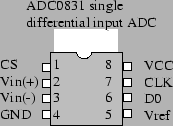The ADC0831 is a differential ADC. This means that it converts the voltage difference between the positive and negative input terminals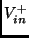and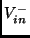on pins 2 and 3, respectively. This voltage difference is denoted as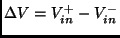. When the control line CS (pin 1) is set low, then the chip begins converting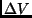into an 8 bit integer. The LSB of this integer equals 1/256 of the voltage between the reference voltage on(pin 5) and ground (pin 4). We usually tie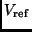to(pin 8) in order to get the full conversion range. As noted above, the conversion is started when the control pin CS (pin 1) is set low. The converted 8 bit integer is then loaded into a buffer that is clock out over the D0 line (pin 6) in synchrony to the clock pulses received on the CLK line (pin 7).

The ADC0831 and the MicroStamp11's SPI port are connected as shown in figure 30. In this case, we connect the ADC0831 pin CS to the MicroStamp11's SS terminal. The MicroStamp11's SRCLK pin is connected to the ADC0831's CLK pin. Since we are reading data from the slave device, the ADC's D0 pin is connected to the MicroStamp11's MISO pin. The voltage to be converted by the ADC0831 lies over the potentiometer shown in figure 30.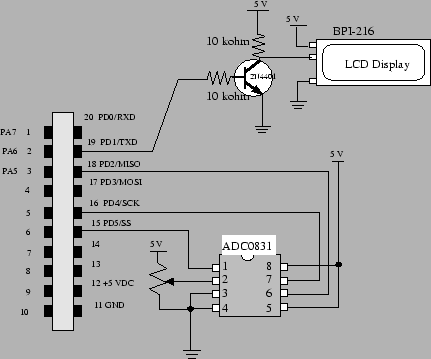Next: Learning Module Exercise Up: Serial Communication Previous: Motorola 68HC11 SPI Interface
Bill Goodwine 2002-09-29